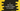# C program to find the total number of digits in a number### C program to find the number of digits in a number :

In this tutorial, we will learn how to find the total number of digits in an integer. For example, the number 123 contains 3 digits. We will learn two different ways to solve this problem. First one is by using a while loop and then secondly, by using a recursive method.

### Algorithm :

The main algorithm or process we are going to use is as below :

• For example, we are finding the number of digits in 234.
• Suppose, count holds the total number of digits. In the beginning, count = 0.
• Divide 234 by 10 . 234/10 = 23. Increment count -> count = 1
• Divide 23 by 10 . 23/10 = 2. Increment count -> count = 2
• Divide 2 by 10 . 2/10 = 0. Increment count -> count = 3
• Since last result was 0, final result is 3 .

### Find the number of digits using a while loop :

Following C program will find the total number of digits using one while loop :

``````#include<stdio.h>

int main(){
//1
int no;
int totalDigits = 0;

//2
printf("Enter a number : ");
scanf("%d",&no);

//3
while(no!=0){
//4
no = no/10;
totalDigits ++;
}

//5
printf("Total digits in the number is %d\n",totalDigits);
}``````

### Explanation :

The commented numbers in the above program denote the step number below :

1. Create one variable no to store the number and one variable totalDigits to store the count of digits in the number.
2. Ask the user to enter a number. Read and save it in variable no.
3. Run one while loop. This loop will run continuously till the number becomes 0.
4. Inside the loop, divide the no by 10 and set this value to no itself. Increment the totalDigits by one.
5. After the loop is completed, totalDigits should hold the total count of digits. Print it out.

### Using recursive method :

In this program, we will use the same approach as above. Only difference is that instead of a while loop, one method will call itself again and again.

``````#include<stdio.h>

//2
int findTotalDigits(int no){
//3
if(no == 0){
return 0;
}

//4
return 1 + findTotalDigits(no/10);
}

int main(){
int no;
int totalDigits = 0;

printf("Enter a number : ");
scanf("%d",&no);

//1
totalDigits = findTotalDigits(no);

printf("Total digits in the number is %d\n",totalDigits);
}``````

### Explanation :

1. In this program, findTotalDigits() function is used to fing the total count. It returns the total count and we are storing it in totalDigits variable.
2. findTotalDigits() takes one integer as input and return one integer (total count).
3. If the provided number is 0, return 1.
4. Else return 1 + findTotalDigits(no/10) , i.e. call the same method again.
5. For number 123 , it will return 1 + findTotalDigits(12) .
6. findTotalDigits(12) will return 1 + findTotalDigits(1).
7. findTotalDigits(1) will return 1 + findTotalDigits(0).
8. findTotalDigits(0) will return 0 ( as mentioned on step 3). So, finally it will rreturn 1 + 1 + 1 = 3 for 123.

### Sample Outputs :

``````Enter a number : 123
Total digits in the number is 3

Enter a number : 1234567
Total digits in the number is 7

Enter a number : 889383
Total digits in the number is 6``````

You might also like :